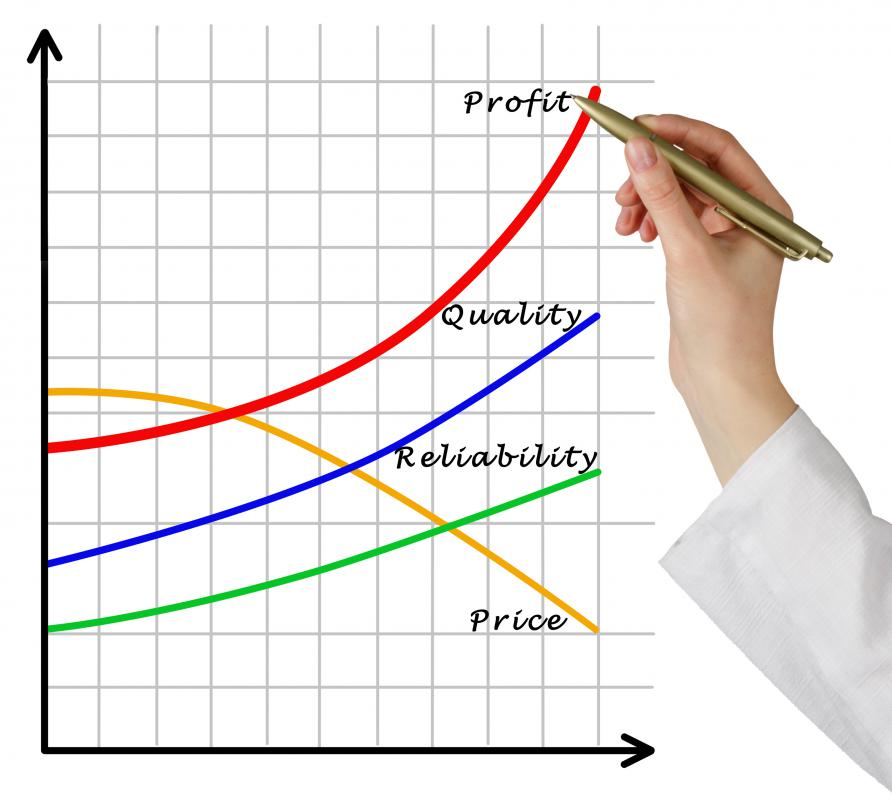# What are the Best Tips for Calculating Profit?

Alexis W.

The best tips for calculating profit depend heavily on the reason for the calculation and what type of calculation is being done. Profit is simply the proceeds made on a given transaction. Individuals can calculate profit of a business venture, profit from working their jobs, or even profit from something such as a garage sale or buying and reselling an item in an online auction. Corporations can calculate profit to determine how successful a company is, to determine how much is made on a given product, or to report profit and loss statements in required disclosures to the government or securities and exchange commission. There are some similarities associated with the way profit is calculated in all these situations, but some differences as well, both in the method of calculating profit and in the best tips for calculating profit that apply in each situation.

In nearly every situation, one of the best tips for calculating profit is to keep careful records. Profit is equal to the money made minus the costs incurred. If a business wants to calculate profit on a product, it must calculate the cost of how much it can sell the product for minus the cost to produce the product. If an individual wants to calculate profit on a business transaction, that person too must subtract cost from money made.

In order to make this calculation successfully, it is essential to keep careful records both of how much all costs were in making the money, and to keep careful records of how much money was actually made. For example, when making a product, there may be ten different raw materials that go into its production. A company that wishes to calculate profit would need to have a record of how much each of these materials cost and what percentage of each material was used in the production of the given product.

Another of the best tips for calculating profit involves knowing the difference between gross profit and net profit. To calculate gross profit, the cost of the production or purchase of the good is subtracted from the money made on the good. No other deductions for income taxes or other overhead expenses are taken out.

To calculate net profit, on the other hand, all deductions must be taken to determine how much actual money goes into the pocket of the company or individual as a result of the transaction. Understanding the difference between net and gross profit is one of the best tips for calculating profit. The two numbers can look very different, and net profit can often be a more accurate estimation of how profitable a given transaction really was.Calculating profit requires understanding the difference between gross profit and net profit.